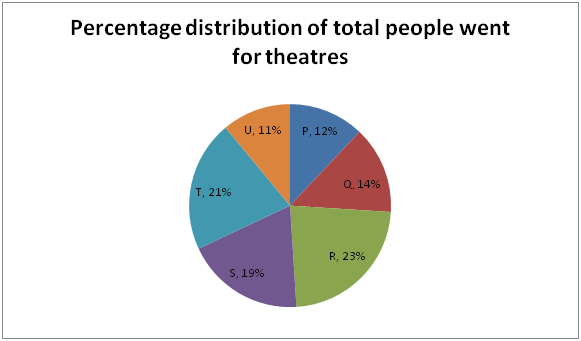# NIACL AO Mains– Quantitative Aptitude Questions Day- 04

Dear Readers, Bank Exam Race for the Year 2019 is already started, To enrich your preparation here we have providing new series of Practice Questions on Quantitative Aptitude – Section. Candidates those who are preparing for NIACL AO Mains 2019 Exams can practice these questions daily and make your preparation effective.

[WpProQuiz 5075]

Directions (Q. 1 – 5): What value should come in the place of question mark (?) in the following questions?

1) 24 × 4140 ÷ 12 % of 2300 = 46 × 990 ÷ 15 – ? – 2400

a) 142

b) 254

c) 276

d) 228

e) None of these

2) (2 (4/5)) ÷ (3 (2/3)) × (1331/1050) = (3/6) of (12/5) of (2178/540) of (?)

a) 1/5

b) 2/7

c) 3/25

d) 5/14

e) None of these

3) 1617 ÷ 11 + 4875 ÷ 13 + 1404 ÷ 9 = (?) % of 150

a) 378

b) 325

c) 423

d) 452

e) None of these

4) 127 % of 1300 + (5/7) of 6895 = (?) + 235

a) 5892

b) 6341

c) 6137

d) 5525

e) None of these

5) 11 (2/3) – 7 (5/6) + 12 (1/4) – 5 (4/9) =? + 3 (7/12)

a) 12 (5/7)

b) 11 (2/9)

c) 7 (1/18)

d) 4 (3/11)

e) None of these

Directions (Q. 6 – 10): Study the following information carefully and answer the given questions:

The following pie chart shows the percentage distribution of total number of people went for a 6 different theatres in a certain city in a day.

Total people (Male + Female) = 120006) Find the ratio between the total number of male went for theatre P to that of total number of people went for theatre R, if the ratio between the total number of male to that of female went to the theatre P is 5 : 4?

a) 20: 69

b) 12: 53

c) 31: 69

d) 74: 133

e) None of these

7) Find the total number of females went for theatre S, if the total number of females went for theatre S is 120 more than the half of the total number of people went for theatre S?

a) 1350

b) 1440

c) 1260

d) 1520

e) None of these

8) Find the ratio between the total number of females went for theatre Q to that of total number of males went for theatre T, if the total number of males went for theatre Q is one-third of the total number of people went for theatre Q and the percentage of total number of females went for theatre T is 45%?

a) 52: 117

b) 66: 101

c) 31: 75

d) 80: 99

e) None of these

9) Total number of people went for theatre R is approximately what percentage of total number of people went for theatre U?

a) 178 %

b) 209 %

c) 154 %

d) 166 %

e) 192 %

10) Total number of females went for theatre R is approximately what percentage more/less than the total number of males went for theatre U, if the percentage of total number of males went for theatre R is 35 % and the total number of females went for theatre U is 600?

a) 150 % less

b) 95 % less

c) 150 % more

d) 120 % less

e) 95 % more

Direction (1-5) :

24 × 4140 ÷ 12 % of 2300 = 46 × 990 ÷ 15 – ? – 2400

24 × 4140 ÷ [(12/100)*2300] = 46 × 990 ÷ 15 – x – 2400

(24*4140)/276 = [(46*990)/15] – x – 2400

X = 3036 – 2400 – 360 = 276

(2 (4/5)) ÷ (3 (2/3)) × (1331/1050) = (3/6) of (12/5) of (2178/540) of (?)

[(14/5) / (11/3)] × (1331/1050) = (3/6)*(12/5)*(2178/540)*x

(14/5)*(3/11)*(1331/1050)*(6/3)*(5/12)*(540/2178) = x

X = 1/5

1617 ÷ 11 + 4875 ÷ 13 + 1404 ÷ 9 = x % of 150

(1617/11) + (4875/13) + (1404/9) = (x/100)*150

147 + 375 + 156 = (3x/2)

678*(2/3) = x

X = 452

127 % of 1300 + (5/7) of 6895 = x + 235

(127/100)*1300 + (5/7)*6895 – 235 = x

X = 1651 + 4925 – 235 = 6341

11 (2/3) – 7 (5/6) + 12 (1/4) – 5 (4/9) – 3 (7/12) = x

(11 – 7 + 12 – 5 – 3) (2/3 – 5/6 + ¼ – 4/9 – 7/12) = x

X = 8 [(48 – 60 + 18 – 32 – 42)/72] = 8 (-68/72) = 8 (-17/18)

X = 127/18 = 7 (1/18)

Direction (6-10) :

The total number of male went for theatre P

= > 12000*(12/100)*(5/9) = 800

The total number of people went for theatre R

= > 12000*(23/100) = 2760

Required ratio = 800: 2760 = 20: 69

The total number of people went for theatre S

= > 12000*(19/100) = 2280

The total number of females went for theatre S

= > (2280/2) + 120 = 1260

The total number of females went for theatre Q

= > 12000*(14/100)*(2/3) = 1120

The total number of males went for theatre T

= > 12000*(21/100)*(55/100) = 1386

Required ratio = 1120: 1386 = 80: 99

Required % = (23/11)*100 = 209 %

Total number of females went for theatre R

= > 12000*(23/100)*(65/100) = 1794

Total number of people went for theatre U

= > 12000*(11/100) = 1320

Total number of males went for theatre U = 1320 – 600 = 720

Required % = [(1794 – 720)/720]*100 = 150 % more